# Simple, Effective Method and Circuit to Measure Very-Low 1/f Voltage Reference Noise (< 1µVP-P, 0.1Hz to 10Hz)

### Abstract

This application note describes a method to measure noise of a precision, low noise voltage reference. This method utilizes two identical voltage references and a differential amplifier to measure ultra-low (0.1Hz to 10Hz) noise of one voltage reference. Using this method, expensive components needed as part of a high-pass filter after voltage reference are eliminated as a high-pass filter is used after the differential amplifier.

Eliminating expensive components needed as part of a high-pass filter after voltage reference can be accomplished by using two identical voltage references and a differential amplifier to measure ultra-low (0.1Hz to 10Hz) noise of one voltage reference.

#### Noise Specs of a Voltage Reference

The voltage noise and temperature drift of a voltage reference usually determine the measurement limits of a data acquisition system (DAS). Voltage reference data sheets generally specify the noise by two separate categories: 1) low-frequency (0.1Hz to 10Hz) as µVP-P; 2) wideband noise as µVRMS for a given band (e.g., 10Hz to 1kHz), or spectral-voltage noise density at a frequency where the noise spectral density has reached its flatness, specified as nV/√Hz .

Data sheets specify low-frequency and wideband noise separately because the latter can be greatly reduced in the system application using lowpass filtering. Filtering of low-frequency noise, however, is cumbersome and not practical, as large capacitors are needed to filter out lower frequencies.

Voltage-reference noise also affects output-voltage accuracy since it is a random signal. For example, if there is 1mVP-P output noise, then for a 3V reference that noise translates into 0.033% voltage randomness which affects the reference's initial accuracy. This application note presents a simple and effective way to measure and reduce low-frequency noise in a voltage reference. The application example aims to achieve low-frequency noise (0.1Hz to 10Hz) below 1µVP-P.

#### Measuring the Noise of a Voltage Reference

A standard way of measuring the noise of a voltage reference is shown in Figure 1. The output of the voltage reference is fed into a highpass filter to pass frequencies of 0.1Hz and above. The highpass filter also performs two beneficial tasks: provides DC-blocking of the voltage reference output, and allows only the AC-signal content above the highpass filter corner frequency to reach the low-noise preamplifier.Figure 1. Typical setup for measuring the noise of a voltage reference.

There are design considerations that affect, even limit, the performance of the above circuit:

1. It requires a large, low-leakage, high-quality, and expensive capacitor to achieve a lower cutoff frequency of the highpass filter.
2. The flicker noise of the input resistor and the input current noise of the low-noise preamplifier add up and determine the low-frequency noise introduced by this resistor; therefore, a smaller resistor will produce lower noise. However, a lower resistance requires a larger capacitance to achieve the cutoff frequency of the highpass filter.
3. The noise introduced by the highpass filter is critical, since it adds to the preamplifier input voltage noise. The total resulting noise at the preamplifier input must be much less than the noise of the voltage reference.

#### Alternative Noise-Measurement Setup

The Figure 2 setup employs two identical voltage references to accurately determine their low-frequency noise. This is an indirect method to measure noise. It operates on the assumption that two different units (from the same manufacturing batch) exhibit very similar noise performance while their noise is uncorrelated.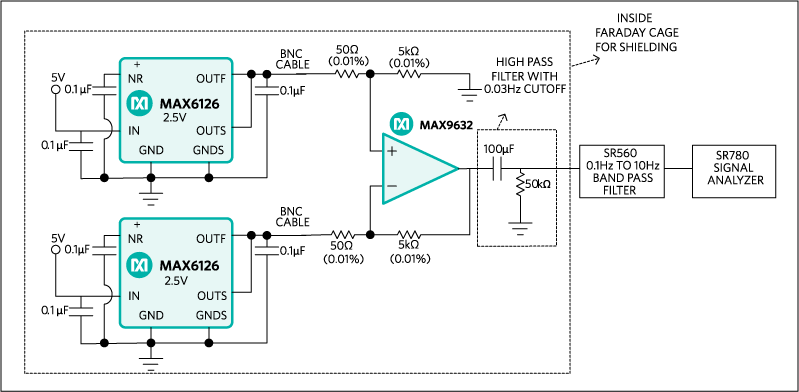Figure 2. Proposed setup to evaluate a voltage reference noise performance.

In our experiment, the setup employs a pair of MAX6126 ultra-low-noise voltage references. The dotted line in the Figure 2 detailed setup shows that all the test circuit is shielded from the outside environment in a Faraday metal cage. Our detailed bench setup can be seen in Figures 6 and 7.

Uncorrelated noise of each voltage reference adds up such that the resulting total noise at the input of the preamplifier can be expressed as in Equation 1:

 eN2 ＝ e12 + e2 (Eq. 1)

where,
eN is the total noise of the two voltage references combined, and
e1, e2 is the noise associated with each voltage reference.

If we assume that the two identical voltage references have the same noise performance, e1 = e2 = e, then Equation 1 yields Equation 2:

 eN ＝ 2 × e (Eq. 2)

To determine the noise contributed by one voltage reference, a correction factor will be used based on the above formula. The total input noise of the differential amplifier in the setup (ein) is calculated with Equation 3. The noise signal e0 incorporates the contributions of the circuit noise sources (excepting the voltage references). It is assumed that all noise sources are uncorrelated:

 ein2 ＝ 2 × e2 + e02 (Eq. 3)

The total input noise is amplified and filtered. The resulting noise signal eout is applied at the input of the spectrum analyzer. This noise signal can be expressed with Equation 4, where G and F are the transfer functions of the differential amplifier and filter, respectively:

 eout2 ＝ G × F × ein2 (Eq. 4)

Using the results of Equations 3 and 4 we can express the setup output noise signal eout as given by Equation 5 below:

 eout2 ＝ G × F × 2 × e2 + G × F × e02 (Eq. 5)

We can separately measure the setup noise by removing the voltage references and connecting the differential amplifier inputs to ground. In that case, the output noise signal eout0 is the result of e0 only. It can be expressed as in Equation 6:

 eout02 ＝ G × F × e02 (Eq. 6)

With the results from Equations 5 and 6 we can calculate the reference noise voltage as shown below in Equation 7:

 E2 ＝(eout2 - eout2)/(2× G × F) (Eq. 7)

The transfer function of the setup (G × F) can be easily evaluated with a network analyzer.

The MAX6126 has a noise-reduction pin NR, where an external capacitor can be connected to ground. This capacitor in conjunction with the on-chip resistor (20kΩ typ.) creates a lowpass filter which reduces the noise of the internal reference. With a 0.1µF noise-reduction capacitor we can filter out spectral components at frequencies above 100Hz. In this application note, we show that a 100µF noise-reduction capacitor can be used to reduce 1/f noise (0.1Hz to 10Hz) since the filter cutoff frequency is ≈ 0.1Hz.

The MAX9632 op-amp has been chosen for its ultra-low noise, both 1/f and wideband. The MAX9632 is used in a differential amplifier configuration. The differential voltage gain is by the ratio of well-matched 5KΩ and 50Ω resistors. These 0.01% matched resistors were chosen to improve the CMRR performance and, consequently, reject unwanted common-mode noise injected by parasitic coupling of external RF and/or AC-line signals. A gain of 100V/V is used, but it can be set higher, if desired. However, the differential amplifier bandwidth BW will be reduced, since BW = GBW/gain.

The output of the differential amplifier is applied to a highpass filter. This filter allows proper setting of the filter-cutoff frequency based on resistor and capacitor values. A combination of 100µF and 50kΩ are used to pass frequencies of 0.03Hz and higher. There are several advantages to using a highpass filter after the low-noise preamplifier. Now we can use generic capacitor and resistor components since their noise is not that critical because the filter is placed after the gain stage. Also, we can customize the filter cutoff frequency as desired. It is important to note that the signal analyzer input is set for DC-coupling mode. Thus, the measurement is not limited by the highpass filter frequency corner of AC-coupling mode in the signal analyzer.

#### Frequency Response of the Signal Chain (Figure 3)

The setup shown in Figure 3 below is used to evaluate the frequency response of the noise measurement setup from Figure 2. In this case, the test signal is applied to one input of the differential amplifier while the other input is connected to ground.Figure 3. Test setup (correspondent to Figure 2) to measure the frequency response.

The frequency response function is G × F (see Equation 7 above). Figure 4 shows that within the low-frequency band (0.1Hz to 10Hz) we may assume that the noise referred to output is gained up by 40dB or 100V/V. Since 0.1Hz and 10Hz are corner frequencies of the external bandpass filter used, they are -3dB points on the gain response.Figure 4. AC gain frequency response (G × F) of the noise measurement setup.

Figure 4 shows that within the band of frequencies (0.1HZ to 10Hz) it is safe to assume that the noise referred to output will be gained up by 40dB or 100V/V. Figure 5 below shows a time-domain output setup for calibration noise. The setup inputs are connected to ground. The output noise is recorded within a 64s time slot, clearly beyond 10 seconds which corresponds to 0.1Hz in frequency domain. This is useful, as it shows that there is little change of the peak-to-peak values of any 10s window within 64 seconds total time.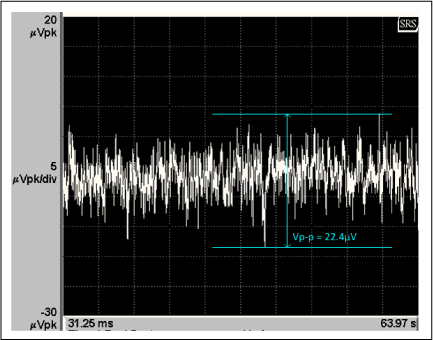Figure 5. Time-domain setup for calibration noise eout0 (see Equation 6).

Figure 6 shows a picture of the bench setup and the equipment used. Figure 7 is a detail of the setup.Figure 6. Picture of the detailed bench setup.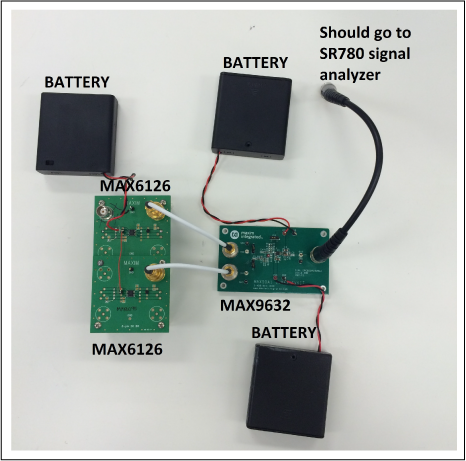Figure 7. Setup inside Faraday cage shown in Figure 6.

#### Voltage Reference Low-Frequency Noise (0.1Hz to 10Hz) Measurement

Figure 8 below shows the time-domain output noise corresponding to two MAX6126 units. The measurement setup is shown in Figure 2 above. Just as for the setup calibration noise, the output noise is recorded within a 64s time slot.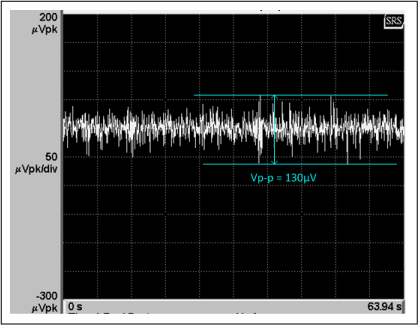Figure 8. Setup for output noise using two MAX6126 units. (Refer to the test setup in Figure 2.)

Equation 7 below is used to calculate the noise contributed by one voltage reference:

e2 ＝ (eout2 - eout02)/(2 × G × F)

√eout2 = 130µVP-P

√eout02 = 22.4µVP-P

G × F = 100V/V or 40dB

Substituting these results in Equation 7 shown above yields: = 0.9055µVP-P The reference noise calculated without the correction term (eout02) is e = 0.919µVP-P. Therefore, the setup calibration noise is negligible compared to the voltage reference noise.

#### The "Bigger" Benefit of a Noise-Reduction (NR) Capacitor for the Voltage Reference

In Figure 9 below, the NR capacitor used in the Figure 2 setup was changed to a 100µF (X5R, 10V, 1206 size) capacitor purchased from Digikey® distribution. This large-value capacitor improves the 0.1Hz to 10Hz noise (Figure 10).Figure 9. MAX6126 noise setup using a 100µF NR capacitor.Figure 10. Output noise of test setup shown in Figure 9.

Equation 7 is used again to calculate the noise contributed by one voltage reference:

e2 ＝ (eout2 - eout02)/(2 × G × F)

√eout2 = 84.6µVP-P

√eout02 = 22.4µVP-P

G × F = 100V/V or 40dB

Substituting these results in Equation 7 shown above yields: = 0.5769µVP-P.

The reference noise calculated without the correction term (eout02) is e = 0.5982µVP-P. Therefore, the setup calibration noise is negligible compared to the voltage reference noise.

#### 0.1Hz to 10Hz Noise Measurement with Competitor Voltage Reference

Figure 11 shows the performance of a competitive voltage reference with the same test setup (see Figure 9) using two of their units. The CLOAD was replaced with 10µF instead of the 0.1µF used in MAX6126 setup. The competitive part exhibits the best noise performance when CLOAD = 10µF.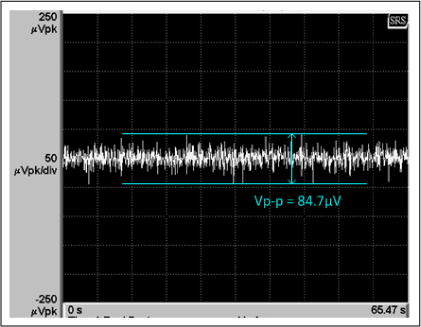Figure 11. Setup output noise using competitive part with CLOAD =10µF.

Equation 7 is used again to calculate the noise contributed by one voltage reference:

e2 ＝ (eout2 - eout02)/(2 × G × F)

√eout2 = 84.7µVP-P

√eout02 = 22.4µVP-P

G × F = 100V/V or 40dB

Substituting these results in Equation 7 yields: e = 0.5776µVP-P. The reference noise calculated without the correction term (eout02) is e = 0.5989µVP-P. Therefore, the setup calibration noise is negligible compared to the voltage reference noise.

#### MAX6126 Output Voltage Temperature Drift with NR Capacitors

We have shown that the 0.1Hz to 10Hz noise of the MAX6126 is significantly reduced when a large-value NR capacitor is used. However, we need to ensure that the reference output-voltage temperature drift is negligibly affected by the leakage of the capacitor (connected between the NR and GND pins). Figure 12 shows MAX6126 temperature drift performance in the following cases: no capacitor, 0.1µF 50V (C0805C104J5RAC7800), and 100µF 10V (C3216X5R1A107M160AC).Figure 12. VOUT(T) –VOUT (25°C): output voltage temperature drift of the MAX6126 for three cases of capacitors on NR pin.

#### Summary

1. The voltage reference outputs are directly connected (no DC blocking required) to the inputs of the differential amplifier. This setup does not need an expensive low-leakage and low-flicker-noise resistor for the highpass filter as required by AC-coupling front-end solutions.
2. In the traditional method (see Figure 1), a highpass filter is required for AC coupling of the reference output voltage to the preamplifier input. The noise associated with the highpass filter components must be much lower than the voltage reference noise to be measured. Also, there is noise voltage developed across the filter resistor by the noise current of the front-end preamplifier input and the capacitor leakage noise current. The input voltage noise of the preamplifier adds up to the above mentioned front-end noise components.
3. The proposed circuit in Figure 2 can measure the ultra-low 1/f frequency noise (< 1µVP-P, 0.1Hz to 10Hz) of a voltage reference by using two identical parts. Also, we assume that the parts exhibit uncorrelated noise. An alternative approach uses a second lower noise voltage reference so that its noise can be easily subtracted from the summed noise due to both references.
4. We have shown that low-frequency noise of the MAX6126 can be substantially reduced by using a 100µF noise-reduction capacitor (connected between NR and GND pins), from 0.9µVP-P to 0.6µVP-P. The downside associated with a large NR capacitor is a much longer power-up settling time (≈ 10 seconds).
5. Finally, the MAX6126 with CNR = 100µF exhibits the same 0.1Hz to 10Hz noise as the competition, but has the advantage of significant power saving since its quiescent current is almost one order of magnitude lower, 0.4mA vs. 5mA.

#### MAX9632 Noise Performance

Figures 13, 14, and 15 illustrate the noise performance of the MAX9632 used as a preamplifier in this application note. This data should show readers why choosing a very-low-noise amplifier is of prime importance to an application.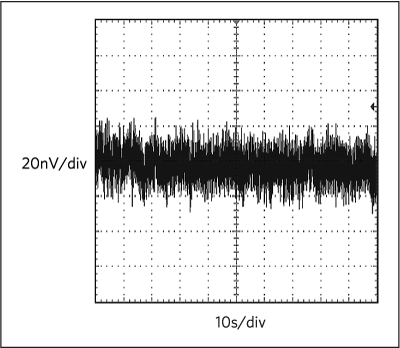Figure 13. The 0.1Hz to 10Hz noise performance of the MAX9632 (see MAX9632 data sheet).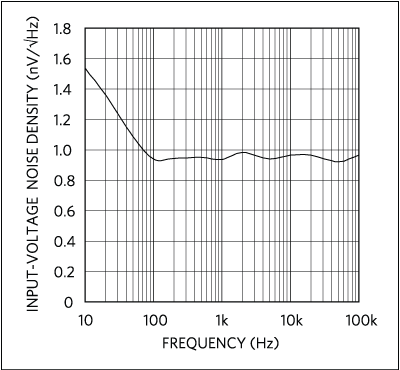Figure 14. Input-voltage noise density performance of the MAX9632 (see MAX9632 data sheet).Figure 15. Input-current noise density performance of the MAX9632 (see MAX9632 data sheet).

This method measures noise of a high-precision, low-noise voltage references using two identical voltage references and a differential amplifier. Using a high-pass filter eliminates expensive components otherwise needed.

A similar version of this App Note appeared in ECN Magazine on August 21, 2015.